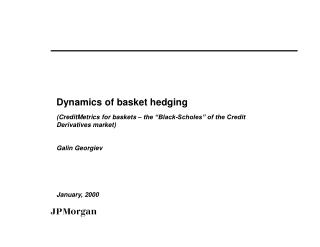Download Presentation# Dynamics of basket hedging - PowerPoint PPT PresentationDownload Presentation- - - - - - - - - - - - - - - - - - - - - - - - - - - E N D - - - - - - - - - - - - - - - - - - - - - - - - - - -
##### Presentation Transcript

1. Dynamics of basket hedging (CreditMetrics for baskets – the “Black-Scholes” of the Credit Derivatives market) Galin Georgiev January, 2000

2. Disclaimer This report represents only the personal opinions of the author and not those of J.P.Morgan, its subsidiaries or affiliates

3. Summary • Definition of a protection contract on an individual name and a first-to-default (FTD) protection contract on two names • The CreditMetrics model for baskets: basic definitions and variations. The basket as a rainbow digital option. • Greeks and dynamic hedging of baskets. Implied vs. realized correlation.

4. Individual protection contract Suppose risk-free interest rates are zero and denote by t the present time. A protection contract maturing at time T on company A entitles the holder to receive \$1 at T if A defaults prior to T (and \$0 otherwise). (In the market place, this is called a zero-coupon credit swap, settled at maturity, with zero recovery). Note that where the latter is the risk-free probability of default of A up to time T, i.e., is proportional to the credit spread of A.

5. FTD protection contract A first-to-default (FTD) protection contract maturing at time T, on companies A and B, entitles the holder to receive \$1 at T if at least one of the companies defaults prior to T (and \$0 otherwise). The price equals the probability of A or B or both defaulting before T.

6. The market perspective While the price of protection for individual credits is (more or less) given by the credit swaps market, there is no liquid market yet for FTD protection (or, equivalently, FTD probability). One needs a model to price as a rainbow derivative on and It depends on the correlation between the underlying spreads in the no-default state and the correlation between the corresponding default events.

7. The CreditMetrics formalism Assumption: where is a univariate random normal variable and is the so-called threshold (defined above; is the cumulative normal distribution). One can be more specific and define where is the normally distributed firm’s asset level and is the (fixed) firm’s liability level (at time T).

8. Asset Distribution at Maturity Default Probability Liability Level Initial Asset Level

9. Inconsistencies of the CreditMetrics model Asset Level Assets <Liabilities => Default Liability Level 1 Liability Level 2 Assets >Liabilities => No Default Time 0 T1 T2

10. Assuming for simplicity constant volatility (of the assets), one can rephrase the price of protection in terms of familiar option theory: where is a standard Brownian motion which we call normalized threshold (with initial point , depending unfortunately on T). This is nothing else but the price of a barrier option (digital) on the underlying struck at 0 and expiring at T.

11. The protection contract as a barrier option We can therefore think of the protection contract as a contingent claim (barrier option) on the underlying Unsurprisingly, it satisfies the (normal version of) the Black-Scholes equation:

12. The FTD protection contract as a rainbow barrier option • The FTD protection price in this context is • which in terms of normalized thresholds means • (where is the bivariate normal cumulative and is the correlation between and ). • For , one has

13. Black-Scholes for the FTD protection • One can easily compute the Greeks and check that our rainbow contingent claim satisfies the two-dimensional version of the (normal) Black-Scholes equation:

14. Hedge ratios • Since the normalized thresholds are not traded, we obviously hedge the FTD protection (the rainbow barrier option) with the two individual protection contracts (1-dim barrier options) and . The corresponding hedge ratios are easily computable:

15. Convexity of the hedged portfolio • The hedged FTD portfolio • is easily seen to have a negative “off-diagonal” convexity: • and positive “diagonal” convexity ( ):

16. Convexity seen through the effect of individual tweaks or parallel tweaks on the hedge ratios of 5 name basket

17. Implied vs. realized correlation • If one buys FTD protection and continuously rehedges, the resulting P&L is • where is the realized correlation and is the bivariate normal density. If , one is long convexity and makes money due to rehedging (but one pays for it upfront because the money earned by selling the original hedges is less).

18. A correlation contract ? • The P&L due to continuous rehedging of the basket is clearly path-dependent. Similarly to the development of the vol contract in standard option theory, the time will come to develop a “correlation contract” whose payoff is path-independent and proportional to realized correlation.